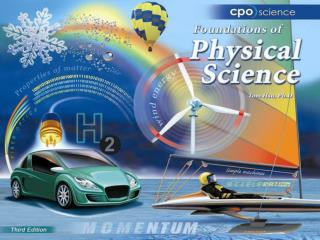Download PresentationChapter Nine: Simple Machines

# Chapter Nine: Simple Machines

Download Presentation## Chapter Nine: Simple Machines

- - - - - - - - - - - - - - - - - - - - - - - - - - - E N D - - - - - - - - - - - - - - - - - - - - - - - - - - -
##### Presentation Transcript

1. Chapter Nine: Simple Machines • 9.1 Types of Simple Machines • 9.2 Mechanical Advantage • 9.3 Levers in the Human Body

2. 9.2 The Lever • A _______ includes a stiff structure (the lever) that rotates around a fixed point called the __________.

3. 9.2 The Lever • Levers are useful because you can arrange the fulcrum and the input arm and output arm to adjust the mechanical advantage of the lever.

4. 9.2 The Lever • Each class of levers is defined by the location of the input and output forces relative to the fulcrum.

5. 9.2 Gears • Many machines require that rotating motion be transmitted from one place to another. • Gears change ________ and _________

6. 9.2 Designing Gear Machines • The gear ratio is the ratio of output turns to input turns. • You can predict how force and speed are affected when gears turn by knowing the number of teeth for each gear.

7. 9.2 Gear Ratios Number of teeth on input gear To=Ni Ti No Turns of output gear Turns of input gear Number of teeth on input gear

8. 9.2 Tension • Ropes and strings carry ________forces along their length. • If the rope is not moving, its tension is equal to the force pulling on each end.

9. 9.2 Ramps • A _________ is a simple machine that allows you to raise a heavy object with less force than you would need to lift it straight up. • The mechanical advantage of a ramp is the ramp length divided by the height of the ramp.

10. 9.2 Screws • A ________is a rotating ramp. • You find the mechanical advantage of a screw by dividing its circumference by the _______.

11. 9.2 Screws • A ________ is like a ramp that can work while in motion (a ramp is always stationary). • A wedge has a side that slopes down to a thin edge. • The mechanical advantage for a wedge is __________ related to the size of the wedge angle.

12. 9.2 Wheel and axle • A _______ rotates around a rod called an _______ • The mechanical advantage is the ratio of the radius of the wheel to the radius of the axle. • The wheel and axle move together to move or lift loads.

13. Solving Problems • A crowbar is a type of lever that you use to pull a nail out of a piece of wood. • If the handle of a crowbar is 40 centimeters and the foot is 2 centimeters, what is its mechanical advantage?

14. Solving Problems • Looking for: • …mechanical advantage of lever • Given • …input arm = 40 cm; output arm = 2 cm • Relationships: • M.A. = Length of input arm Length of output arm • Solution • M.A. = 40 cm ÷ 2 cm = 20